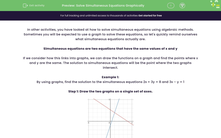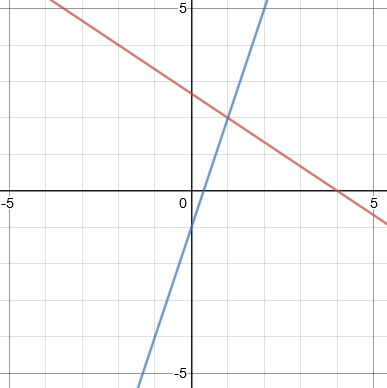# Solve Simultaneous Equations Graphically

In this worksheet, students will practise solving two simultaneous equations (both linear and non-linear) by drawing graphs and looking for the intersections.Key stage:  KS 4

Year:  GCSE

GCSE Subjects:   Maths

GCSE Boards:   AQA, OCR, Pearson Edexcel,

Curriculum topic:   Algebra

Curriculum subtopic:   Solving Equations and Inequalities Algebraic Equations

Popular topics:   Algebra worksheets

Difficulty level:#### Worksheet Overview

In other activities, you have looked at how to solve simultaneous equations using algebraic methods. Sometimes you will be expected to use a graph to solve these equations, so let's quickly remind ourselves what simultaneous equations actually are.

Simultaneous equations are two equations that have the same values of x and y

If we consider how this links into graphs, we can draw the functions on a graph and find the points where x and y are the same. The solution to simultaneous equations will be the point where the two graphs intersect.

Example 1:

By using graphs, find the solution to the simultaneous equations 2x + 3y = 8 and 3x – y = 1

Step 1: Draw the two graphs on a single set of axes.Step 2: Read off the coordinates where the two lines cross.From the graph, we can see that the solutions to the equations are x = 1 and y = 2

Other graphs

Unfortunately, this topic isn’t limited to using linear graphs. You could also be expected to do this with quadratics and circles as well.

The good news is that the method to complete this is exactly the same, you’ll just could get more solutions.

Example 2:

By using graphs, find the solution to the simultaneous equations x2 + y2 = 25 and y = 2x + 1

Step 1: Draw the two graphs on a single set of axes.Step 2: Read off the coordinates where the two lines cross.From this graph, we can see that the intersections are at (-2.6,-4.3) and (1.8,4.7)

This gives two sets of solutions:

x1 = -2.6 and y1 = -4.3

x2 = 1.8 and y2 = 4.7

Let's move on to some questions now.

### What is EdPlace?

We're your National Curriculum aligned online education content provider helping each child succeed in English, maths and science from year 1 to GCSE. With an EdPlace account you’ll be able to track and measure progress, helping each child achieve their best. We build confidence and attainment by personalising each child’s learning at a level that suits them.

Get started